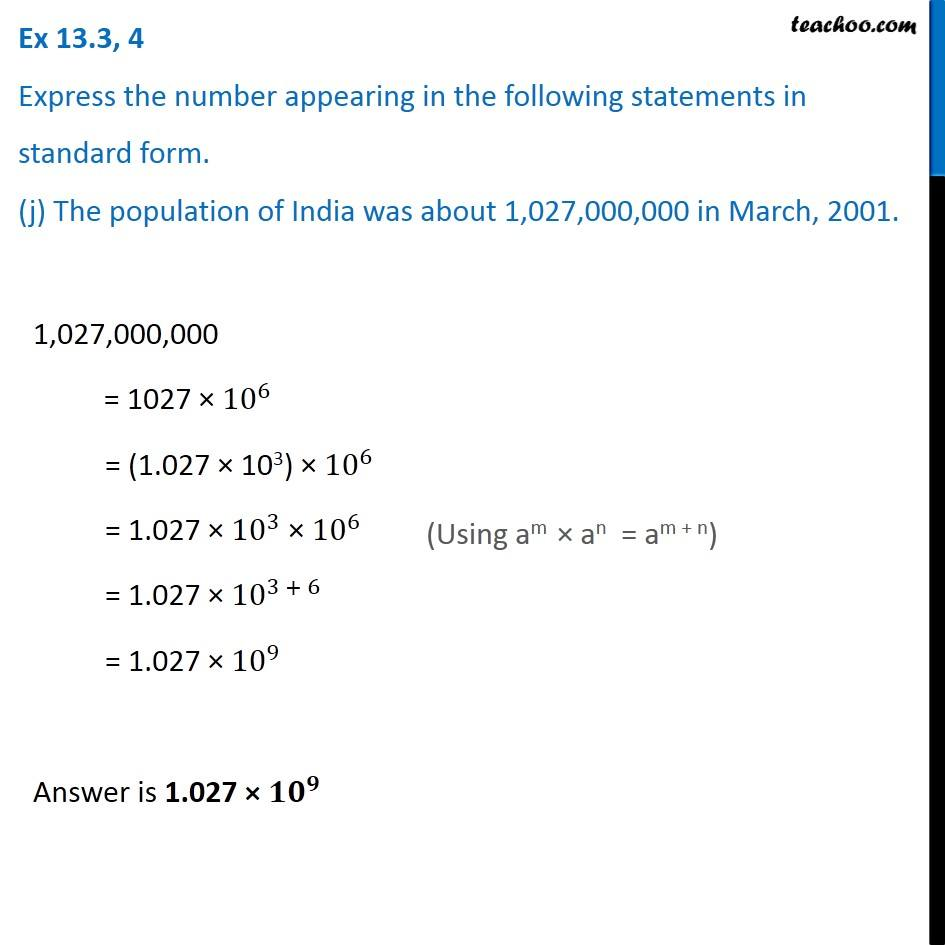Ex 13.3

Chapter 13 Class 7 Exponents and Powers
Serial order wiseIntroducing your new favourite teacher - Teachoo Black, at only ₹83 per month

### Transcript

Ex 13.3, 4 Express the number appearing in the following statements in standard form. (j) The population of India was about 1,027,000,000 in March, 2001. 1,027,000,000 = 1027 × 10^6 = (1.027 × 103) × 10^6 = 1.027 × 10^3 × 10^6 = 1.027 × 10^(3 + 6) = 1.027 × 10^9 Answer is 1.027 × 〖𝟏𝟎〗^𝟗